# 20.11: The Generation of Energy from Carbohydrate Metabolism

$$\newcommand{\vecs}{\overset { \rightharpoonup} {\mathbf{#1}} }$$ $$\newcommand{\vecd}{\overset{-\!-\!\rightharpoonup}{\vphantom{a}\smash {#1}}}$$$$\newcommand{\id}{\mathrm{id}}$$ $$\newcommand{\Span}{\mathrm{span}}$$ $$\newcommand{\kernel}{\mathrm{null}\,}$$ $$\newcommand{\range}{\mathrm{range}\,}$$ $$\newcommand{\RealPart}{\mathrm{Re}}$$ $$\newcommand{\ImaginaryPart}{\mathrm{Im}}$$ $$\newcommand{\Argument}{\mathrm{Arg}}$$ $$\newcommand{\norm}{\| #1 \|}$$ $$\newcommand{\inner}{\langle #1, #2 \rangle}$$ $$\newcommand{\Span}{\mathrm{span}}$$ $$\newcommand{\id}{\mathrm{id}}$$ $$\newcommand{\Span}{\mathrm{span}}$$ $$\newcommand{\kernel}{\mathrm{null}\,}$$ $$\newcommand{\range}{\mathrm{range}\,}$$ $$\newcommand{\RealPart}{\mathrm{Re}}$$ $$\newcommand{\ImaginaryPart}{\mathrm{Im}}$$ $$\newcommand{\Argument}{\mathrm{Arg}}$$ $$\newcommand{\norm}{\| #1 \|}$$ $$\newcommand{\inner}{\langle #1, #2 \rangle}$$ $$\newcommand{\Span}{\mathrm{span}}$$

We will consider here the reverse process of photosynthesis, namely how carbohydrates, especially glucose, are converted to energy by being broken down into carbon dioxide and water.

A general summary of the several stages involved is shown in Figure 20-8. Initially, the storage fuels or foodstuffs (fats, carbohydrates, and proteins) are hydrolyzed into smaller components (fatty acids and glycerol, glucose and other simple sugars, and amino acids). In the next stage, these simple fuels are degraded further to two-carbon fragments that are delivered as the $$\ce{CH_3C=O}$$ group (ethanoyl, or acetyl) in the form of the thioester of coenzyme A, $$\ce{CH_3COS}$$CoA. The structure of this compound and the manner in which fatty acids are degraded has been considered in Section 18-8F, and amino acid metabolism is discussed briefly in Section 25-5C. This section is concerned mainly with the pathway by which glucose is metabolized by the process known as glycolysis.Figure 20-8: Perspective of the metabolic scheme whereby carbohydrates, fats, and proteins in foodstuffs are oxidized to $$\ce{CO_2}$$, showing the link between glycolysis, the citric acid cycle, and oxidative phosphorylation.

In the conversion of glucose to $$\ce{CH_3COS}$$CoA, two carbons are oxidized to carbon dioxide with consumption of two oxygen molecules:

$\ce{C_6H_{12}O_6} + 2 \textbf{CoA} \ce{SH} + \left[ 2 \ce{O_2} \right] \rightarrow 2 \ce{CH_3COS} \textbf{CoA} + 4 \ce{H_2O} + 2 \ce{CO_2} \tag{20-5}$

For further oxidation to occur, the $$\ce{CH_3COS}$$CoA must enter the next stage of metabolism, whereby the $$\ce{CH_3C=O}$$ group is converted to $$\ce{CO_2}$$ and $$\ce{H_2O}$$. This stage is known variously as the citric acid cycle, the tricarboxylic acid cycle, or the Krebs cycle, in honor of H. A. Krebs (Nobel Prize, 1953), who first recognized its cyclic nature in 1937. We can write an equation for the process as if it involved oxygen:

$2 \ce{CH_3COS} \textbf{CoA} + \left[ 4 \ce{O_2} \right] \rightarrow 4 \ce{CO_2} + 2 \ce{H_2O} + 2 \textbf{CoA} \ce{SH} \tag{20-6}$

Notice that combination of the reactions of Equations 20-5 and 20-6, glycolysis plus the citric acid cycle, oxidizes glucose completely to $$\ce{CO_2}$$ and $$\ce{H_2O}$$:

$\ce{C_6H_{12}O_6} + \left[ 6 \ce{O_2} \right] \rightarrow 6 \ce{CO_2} + 6 \ce{H_2O} \tag{20-7}$

But, as you will see, none of the steps uses molecular oxygen directly. Hence there must be a stage in metabolism whereby molecular oxygen is linked to production of oxidizing agents that are consumed in glycolysis and in the citric acid cycle.

The coupling of oxygen into the metabolism of carbohydrates is an extremely complex process involving transport of the oxygen to the cells by an oxygen carrier such as hemoglobin, myoglobin, or hemocyanin. This is followed by a series of reactions, among which $$\ce{NADH}$$ is converted to $$\ce{NAD}^\oplus$$ with associated formation of three moles of ATP from three moles of ADP and inorganic phosphate. Another electron-carrier is flavin adenine dinucleotide ($$\ce{FAD}$$; Section 15-6C), which is reduced to $$\ce{FADH_2}$$ with an associated production of two moles of ATP from two moles of ADP. These processes are known as oxidative phosphorylation and can be expressed by the equations: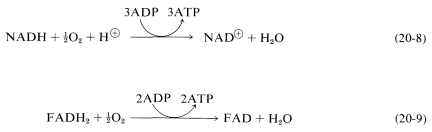Oxidative phosphorylation resembles photophosphorylation, discussed in Section 20-9, in that electron transport in photosynthesis also is coupled with ATP formation.

By suitably juggling Equations 20-7 through 20-9, we find that the metabolic oxidation of one mole of glucose is achieved by ten moles of $$\ce{NAD}^\oplus$$ and two moles of $$\ce{FAD}$$:The overall result is production of 36 moles of ATP from ADP and phosphate per mole of glucose oxidized to $$\ce{CO_2}$$ and $$\ce{H_2O}$$. Of these, 34 ATPs are produced according to Equation 20-10 and, as we shall see, two more come from glycolysis.

## Glycolysis

Glycolysis is the sequence of steps that converts glucose into two $$\ce{C_3}$$ fragments with the production of ATP. The $$\ce{C_3}$$ product of interest here is 2-oxopropanoate (pyruvate):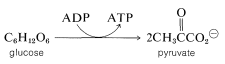There are features in this conversion that closely resemble the dark reactions of photosynthesis, which build a $$\ce{C_6}$$ chain (fructose) from $$\ce{C_3}$$ chains (Section 20-9). For example, the reactants are either phosphate esters or mixed anhydrides, and the phosphorylating agent is ATP:

$\ce{ROH} + \text{ATP} \rightarrow \ce{RO-PO_3^2-} + \text{ADP} + \ce{H}^\oplus$

Furthermore, rearrangements occur that interconvert an aldose and ketose,and the cleavage of a $$\ce{C_6}$$ chain into two $$\ce{C_3}$$ chains is achieved by a reverse aldol condensation:Also, oxidation of an aldehyde to an acid is accomplished with $$\ce{NAD}^\oplus$$. There is a related reaction in photosynthesis (Section 20-9) that accomplishes the reduction of an acid to an aldehyde and is specific for $$\ce{NADPH}$$, not $$\ce{NADH}$$: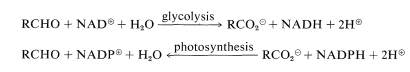Figure 20-9 and each of the steps is identified more specifically in the ensuing discussion.Figure 20-9: The glycolytic sequence. The dashed arrows in the reverse direction indicate the steps in the synthesis of glucose from pyruvate (gluconeogenesis) that differ from those in glycolysis.

First, glucose is phosphorylated to glucose 6-phosphate with ATP. Then an aldose $$\rightleftharpoons$$ ketose rearrangement converts glucose 6-phosphate into fructose 6-phosphate. A second phosphorylation with ATP gives fructose 1,6-diphosphate:At this stage the enzyme aldolase catalyzes the aldol cleavage of fructose 1,6-diphosphate. One product is glyceraldehyde 3-phosphate and the other is 1,3-dihydroxypropanone phosphate. Another ketose $$\rightleftharpoons$$ aldose equilibrium converts the propanone into the glyceraldehyde derivative:The next step oxidizes glyceraldehyde 3-phosphate with $$\ce{NAD}^\oplus$$ in the presence of phosphate with the formation of 1,3-diphosphoglycerate:The mixed anhydride of phosphoric acid and glyceric acid then is used to convert ADP to ATP and form 3-phosphoglycerate. Thereafter the sequence differs from that in photosynthesis. The next few steps accomplish the formation of pyruvate by transfer of the phosphoryl group from $$\ce{C_3}$$ to $$\ce{C_2}$$ followed by dehydration to phosphoenolpyruvate. Phosphoenolpyruvate is an effective phosphorylating agent that converts ADP to ATP and forms pyruvate: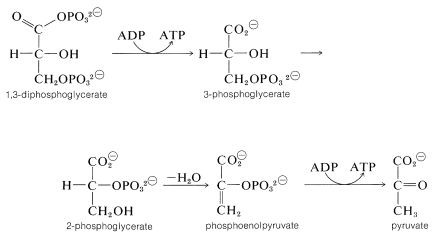The net reaction at this point produces more ATP than is consumed in the phosphorylation of glucose and fructose.

What happens thereafter depends on the organism. With yeast and certain other microorganisms, pyruvate is decarboxylated and reduced to ethanol. The end result of glycolysis in this instance is fermentation. In higher organisms, pyruvate can be stored temporarily as a reduction product (lactate) or it can be oxidized further to give $$\ce{CH_3COS}$$CoA and $$\ce{CO_2}$$. The $$\ce{CH_3COS}$$CoA then enters the citric acid cycle to be oxidized to $$\ce{CO_2}$$ and $$\ce{H_2O}$$, as discussed in the next section:## The Citric Acid (Krebs) Cycle

Glycolysis to the pyruvate or lactate stage liberates heat, which can help keep the organism warm and produce ATP from ADP for future conversion into energy. However, glycolysis does not directly involve oxygen and does not liberate $$\ce{CO_2}$$, as we might expect from the overall process of the metabolic conversion of glucose to carbon dioxide and water (Equation 20-10). The liberation of $$\ce{CO_2}$$ occurs subsequent to pyruvate formation in a process called variously, the citric acid cycle, the Krebs cycle, or the tricarboxylic acid (TCA) cycle.Figure 20-10, and it will help to refer to this diagram as each of the steps in it are discussed in more detail.

The initial step, which is not really part of the cycle, is conversion of pyruvate to ethanoyl CoA (acetyl CoA):

To achieve the oxidation of acetyl CoA on a continuing basis, intermediates consumed in certain steps must be regenerated in others. Thus we have a situation similar to that in the Calvin cycle (Section 20-9), whereby the first stage of the cycle achieved the desired reaction ($$\ce{CO_2}$$ formation) and the second stage is designed to regenerate intermediates necessary to perpetuate the cycle.

The entry point is the reaction between acetyl CoA and a four-carbon unit, 2-oxobutanedioic acid. An aldol-type addition of the $$\ce{CH_3CO}$$ group to this $$\ce{C_4}$$ keto acid extends the chain to a branched $$\ce{C_6}$$ acid (as citric acid):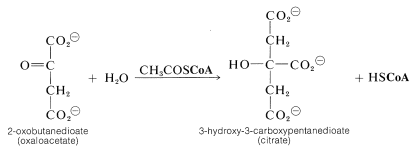Dehydration-rehydration of citrate converts it to isocitrate: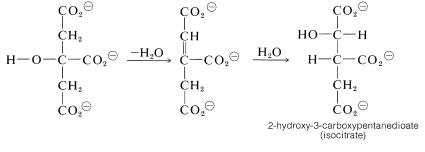From here, oxidation of the hydroxyl function with $$\ce{NAD}^\oplus$$ gives a keto acid, which loses $$\ce{CO_2}$$ readily (Section 18-4) and affords 2-oxopentanedioate:We now have a $$\ce{C_5}$$ keto acid that can be oxidized in the same way as the $$\ce{C_3}$$ keto acid, pyruvic acid, to give a butanedioyl CoA: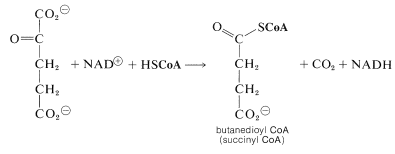Two molecules of $$\ce{CO_2}$$ now have been produced and the remaining part of the citric acid cycle is concerned with regeneration of the CoA for forming acetyl CoA from 2-oxopropanoate, and also with regenerating the 2-oxobutanedioate, which is the precursor of citrate. The steps involved are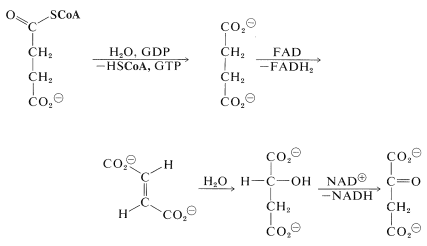The hydrolysis of the acyl CoA in the first step is used for energy storage by conversion of guanosine diphosphate (GDP) to guanosine triphosphate (GTP):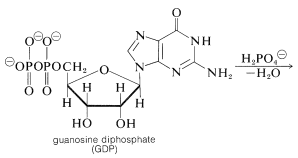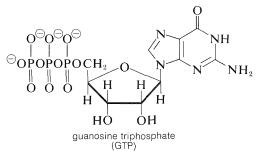The hydration of the trans-butenedioate (Section 10-3G) and the final oxidation reaction (Section 15-6C) have been discussed previously.

## Alternative Routes in Carbohydrate Metabolism

There is an alternative route, called the pentose phosphate pathway, by which glucose enters the glycolytic sequence to pyruvate. This route achieves the oxidative decarboxylation of glucose to give ribose, as the 5-phosphate ester. The essential steps areFigure 20-11). The reactions that accomplish this are very similar to those of the Calvin cycle (Section 20-9), only in reverse. They may be summarized as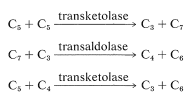The net result is that three pentoses are converted into two molecules of fructose and one of glyceraldehyde $$\left( 3 \ce{C_5} \rightarrow 2 \ce{C_6} + \ce{C_3} \right)$$.Figure 20-11: Schematic representation of metabolism of glucose by way of glycolysis and citric acid cycle, and the pentose phosphate, lactate, and gluconeogenesis links.

The relationship of the pentose-phosphate pathway to glycolysis is shown in Figure 20-11. The steps involved in the pentose shunt are readily reversible, but there are several steps in glycolysis that are not. These are the phosphorylation steps (Figure 20-9). Yet, there has to be a return route from pyruvate to glucose. This route is called gluconeogenesis and, in animals, takes place in the liver. We shall not discuss the steps in gluconeogenesis except to indicate again that they are not all the reverse of glycolysis. For comparison, the steps that differ are indicated in Figure 20-9 by dashed lines.

Why is lactate formed from pyruvate in the metabolism of glucose? Pyruvate $$+ \: \ce{NADH} + \ce{H}^\oplus \rightarrow$$ lactate $$+ \: \ce{NAD}^\oplus$$ is a dead-end path, but it does furnish the $$\ce{NAD}^\oplus$$ needed for glycolysis in active muscle. This route for forming $$\ce{NAD}^\oplus$$ is important, because in circumstances of physical exertion, the rate of production of $$\ce{NAD}^\oplus$$ from oxidative phosphorylation may be slower than the demand for $$\ce{NAD}^\oplus$$, in which case a temporary supply is available from the pyruvate $$\rightarrow$$ lactate reduction. The lactate so formed builds up in muscle tissue under conditions of physical exertion and is apt to cause muscles to "cramp". The excess lactate so formed ultimately is removed by being converted back to pyruvate by oxidation with $$\ce{NAD}^\oplus$$.

The beauty of the metabolic cycle through pyruvate, shown in summary in Figure 20-11, is the way it can be tapped at various points according to whether the organism requires ATP (from glycolysis), $$\ce{NADH}$$ (from pentose shunt), or $$\ce{NAD}^\oplus$$ (from the lactate siding).